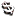Post# of 2456ChevyRe: 56Chevy post# 7

Saturday, 10/13/2012 2:19:42 AM

Comparison Data - Impressive trends!

Financial performance highlights for the three months ended June 30, 2012 as compared to the three months ended June 30, 2011:

• Earnings growth of 195%
• Return on average assets of 1.30%, an increase of 189%
• Non-interest income growth of 79%
• Non-interest expense reduction of 10%
• Cost of funds reduced 22%
Loan growth of 19%
Nonperforming assets reduced 75%, from 1.40% to 0.34% of total assets
• Non-interest bearing deposit growth of 30%
• A fortress balance sheet, with a tier 1 leverage ratio of 13.38% and total risk based capital ratio of 22.07%
No TARP

Financial performance highlights for the three months ended June 30, 2012 as compared to the three months ended March 31, 2012:

Earnings growth of 74%
• Non-interest income growth of 19%
• Non-interest expense reduction of 8%
Nonperforming assets reduced 57%, from 0.83% to 0.34% of total assets
• Asset growth of 5%
Loan growth of 8%
• Non-interest bearing deposit growth of 12%
• Total deposit growth of 7%

========================

Financial performance highlights for the three months ended March 31, 2012 as compared to the three months ended March 31, 2011:

• Earnings growth of 53%
• Nonperforming assets reduced 50%
• Non-interest bearing deposit growth of 38%
• Non-interest income growth of 14%
• Non-interest expense reduction of 10%
• Cost of funds reduced 13%
• Loan growth of 2.0%
• A fortress balance sheet, with a tier 1 leverage ratio of 13.64% and total risk based capital ratio of 22.60%
• No TARP

Financial performance highlights for the three months ended March 31, 2012 as compared to the three months ended December 31, 2011:

• Earnings growth of 45%
• Nonperforming assets reduced 36%
• Non-interest bearing deposit growth of 12%
• Non-interest income growth of 83%
• Asset growth of 5.0%
• Total deposit growth of 5.0%

*Good candidate to be acquired.

Learn something new every time you invest...and take it with you to the next opportunity.

 Volume: Day Range: Bid: Ask: Last Trade Time: Total Trades:
• 1D
• 1M
• 2M
• 3M
• 6M
• 1Y
• 2Y
• 3Y
• 5Y